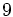# Problems in elementary group theory/solutions

This is the solutions page to its parent page.

## Definition understanding

### True/False problems

1. False: A group must contain an identity element, and is hence always nonempty. Relevant terms: group
2. True: The map sends the unique element in the trivial group to the identity element of the group. The category-theoretic interpretation is that the trivial group is an initial object in the category of groups. Relevant terms: trivial group, homomorphism of groups
3. True: Set-theoretically, the map sends all elements of the group to the single element of the trivial group. The homomorphism conditions are readily checked. In categoryRelevant terms: homomorphism of groups, trivial group
4. False: Consider the group of integers (under addition) and the subset comprising nonnegative integers. This is not a subgroup, since it does not have additive inverses. However, the result is true for finite groups, and a slightly modified version is true for arbitrary groups. Relevant terms: subgroup Relevant facts: sufficiency of subgroup condition
5. True: This boils down to proving uniqueness of identity element and inverses. Relevant facts: equivalence of definitions of group
6. False: The inverse map and identity element store practically no information about the group structure. In fact, the only information the inverse map carries about the group structure, is whether an element has order two.
2. False: The elementary Abelian group of order$9$ is a union of four proper subgroups of order three.
4. False: The result would be true if we assumed$\varphi$ to be surjective. Relevant terms: normal subgroup Relevant facts: normality is image-closed